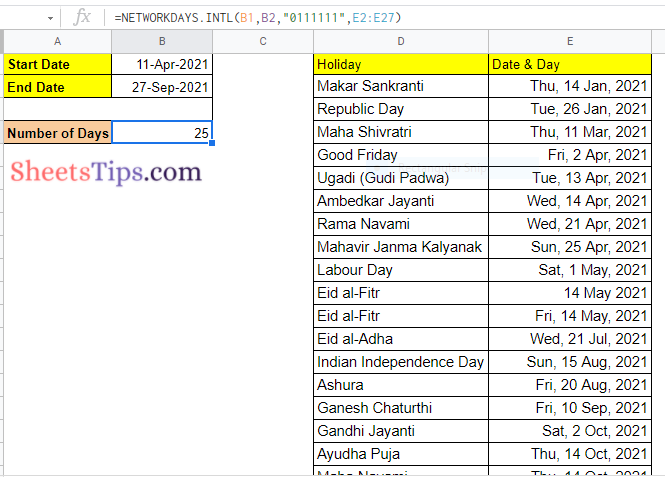# How to Calculate Days Between Two Dates in Google Sheets (With Examples)

With the help of Google Sheets, we can easily work with data and time functions and analyze the data in real-time. For example, if you want to calculate the number of days in between two dates, then you can easily perform the same with the help of Google Sheets without doing any manual calculations.

## How to Calculate Total Days Between Two Dates?

Let us say you are working on a project and your manager has said that you will be working on this project from this date to this date. You can make use of Google Sheets to know how many days you will be working on this project. For this follow the steps as listed below:

• Step 1: In the Google Spreadsheet, enter the start date of your project in Cell B1.
• Step 2: In Cell B2, enter the end date of your project.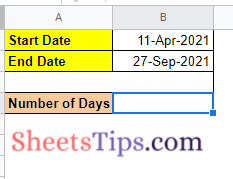• Step 3: Now move to Cell B4 to know how many days you will be working on this project and enter the formula “=B2-B1”.
• Step 4: Press the “Enter” button.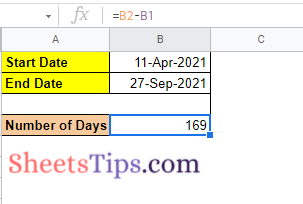Now you will see the results. This calculation is quite simple since we have just used the minus operator to know the number of days between two dates.

In this example, we have calculated the in-between dates between the start and the end date. For example, if you want to calculate the total number of days including the project start and end date, then you can simply replace this formula “=B2-B1+1” instead of “=B2-B1”

### How to Calculate Number of Workdays Between Two Days?

Now let us say if you have Week-Offs on Saturday and Sunday. So if you want to calculate the number of working days excluding your week off from the given two dates, you can simply follow the steps as given below:

• Step 2: In Cell B2, enter your project end date.
• Step 3: Now move to the B4 cell and enter the formula as “=NETWORKDAYS(B1, B2)”.
• Step 4: Press the “Enter” button and you will see the results in Cell B4.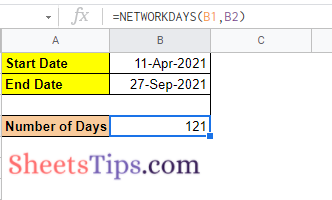### How to Calculate the Workdays Excluding Public Holidays?

The public or government holidays vary from country to country. So if you want to calculate the number of days excluding the public holidays in your project, you will need to list down the number of public holidays in a separate cell.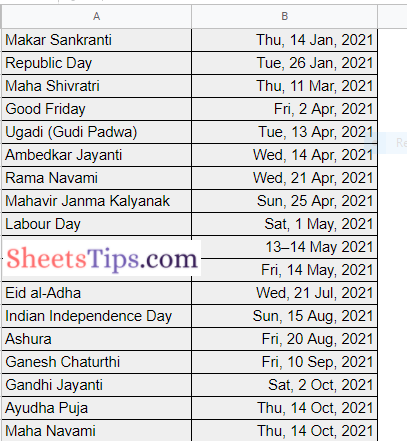After listing out the public holidays, follow the steps given below to calculate the workdays excluding the public holidays.

• Step 1: In Cell B1, enter your project start date.
• Step 2: In Cell B2, enter your project end date.
• Step 3: Enter the number of public holidays in proper date format in the same sheet as shown in the image.
• Step 4: Now move to Cell B4 and enter the formula as =NETWORKDAYS(B1,B2,E2:E27).
• Step 5: Press the “Enter” button and you will results.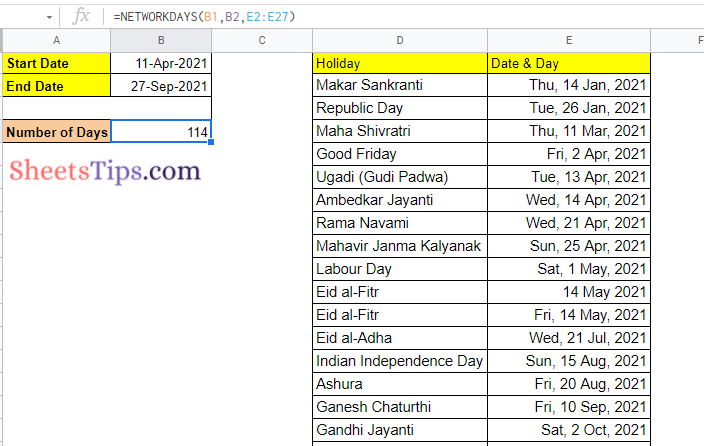### How to Calculate the Workdays when Weekoffs are not Saturday and Sunday?

The Network function in Google Sheets will automatically assume that the week offs are Saturday and Sunday. However, the week off days may vary from country to country and also depending upon the project you are working on. In such cases, you can simply follow the steps given below to calculate the workdays between two dates.

• Step 1: Enter the start date of your project in Cell B1.
• Step 2: Enter the end date of your project in Cell B2.
• Step 3: Now in Cell B4, enter the formula =NETWORKDAYS.INTL(B1,B2,7,E2:E27).Step 4: Press the enter key. You will see results.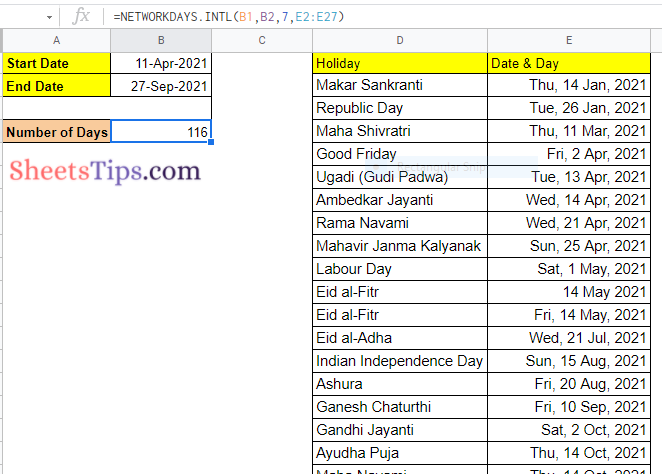In the above formula, 7 represents Friday and Saturday as week off.

Refer to the list below to know the week off days based on the arguments. You can alter the formula using the arguments given below:

• 1 = Saturday/Sunday are weekend days
• 2 = Sunday/Monday are weekend days
• 3 = Monday/Tuesday are weekend days
• 4 = Tuesday/Wednesday are weekend days
• 5 = Wednesday/Thursday are weekend days
• 6 = Thursday/Friday are weekend days
• 7 = Friday/Saturday are weekend days
• 11 = Sunday is the only weekend day
• 12 = Monday is the only weekend day
• 13 = Tuesday is the only weekend day
• 14 = Wednesday is the only weekend day
• 15 = Thursday is the only weekend day
• 16 = Friday is the only weekend day

### How to Calculate Workdays with Non-Consecutive Off Days?

Assume you have a job that only requires you to work on certain days of the week (say, Monday, Tuesday, and Thursday).

Counting the number of working days between two dates gets considerably more difficult with this setup.

Thankfully, this was taken into consideration when the NETWORKDAYS.INTL function was created.

You must explicitly define which days are functioning and which are not when using the NETWORKDAYS.INTL method for something like this.

And you do that by utilising a string of seven digits in a row (where each number is either 1 or 0). The seven days of the week are represented by these numerals. Monday is represented by the first number in the series, Tuesday by the second, and so on.

It’s a working day if the number is zero, and it’s a non-working day if the number is one. So 0000011 denotes that the first five days of the week (Monday through Friday) are working days, while Saturday and Sunday are weekend days (or non-working days)

You may now build whatever week you like with a mix of working and non-working days. These don’t have to be in any particular order.

In our case, the code would be 0010111 because Monday, Tuesday, and Thursday are working days.

Also, as shown below, you may use this code in the NETWORKDAYS.INTL function:

=NETWORKDAYS.INTL(B1,B2,”0010111″,E2:E27)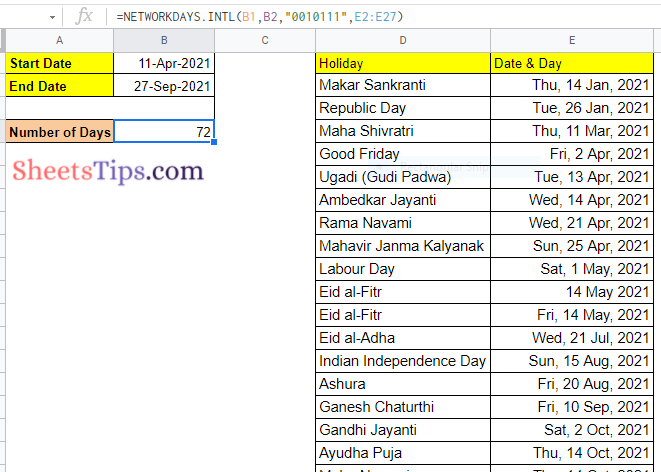### How to Calculate all Mondays between Two Dates?

You may just need to calculate the number of one single weekday rather than the number of working days.

Let us say you want to figure out how many Mondays there are in a certain calendar span (while also accounting for holidays).

This might be useful if you want to determine how many update calls will be made within the specified periods if the call takes place on Mondays.

Assume you have the following data collection and wish to calculate the number of Mondays between the start and end dates:

I will use the NETWORKDAYS.INTL function with a string of seven consecutive integers that indicate the days of the week to determine the number of Mondays.

This is only a variant of the previous example in which we calculated non-consecutive working days. We just need to identify Monday as the working day in this case, and the rest of the days are all weekend days.
As a result, the string of digits would be “0111111,” with 0 representing a Monday working day.

The formula below will calculate the number of working Mondays between the start and finish dates (while taking into account holidays):

=NETWORKDAYS.INTL(B1,B2,”0111111″,E2:E12)